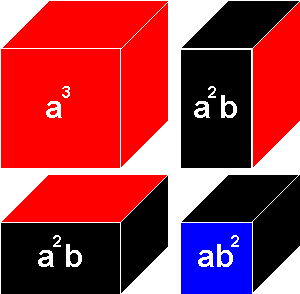# Binomial cube

<videoflash>D9Bvi00xlns&NR</videoflash>

## Contents

3-6

### Materials

A box containing eight wooden blocks:

• One red cube
• One blue cube
• Three red and black rectangular prisms
• Three blue and black rectangular prisms

All the blocks fit into a natural wood box. Each side of the cube has the same dimensions and pattern, and represents the square of (a + b). The faces of the small blocks are color coded: a2 is always red, b2 is always blue, and "ab" is always black.

a2 + 2ab + b2 = (a + b)2

### Preparation

Algebraic expression: (a + b)3 = (a + b)(a + b)(a + b) = a3+3a2b+3ab2+b3

This is an individual exercise (Note: work cycle to be observed)

The child takes the cube apart beginning with a3 and lays out the pieces as shown, according to the formula. The child reconstructs the cube, matching red faces, black faces, and blue faces, beginning with a3.

First Layer of the Binomial Cube: (represents a3+2a2b+ab2)

###Second layer of the Binomial Cube: (represents a2b+2ab2+b3)

Adding both layers together, we get the following: (a3+3a2b+3ab2+b3)

### Presentation

1. The Directress shows the child how to carry the box to the table. She sits besides the child, open up the box and lays out the blocks in the following pattern that will make it easy  for rebuilding the cube in two layers.
2. The Directress then shows the child how to rebuild the cube. The colors printed on the lid act as a guide.

### Control Of Error

The color codes.
The cube can not be built if incorrectly assembled.

### Points Of Interest

At this sensorial stage, the child is not given the plan.

### Purpose

• Provide the child with tangible experience of the way in which the cube can be divided and sub-divided.
• Lay the foundations for the later study of algebra.
• The child is at the stage of the absorbent mind. The child is not asked to understand the formula, but is using the cube in a mathematical way. The child will build up a predisposition to enjoy and understand mathematics later.